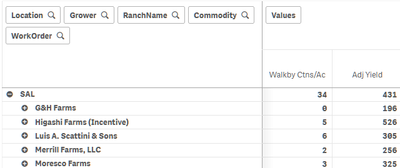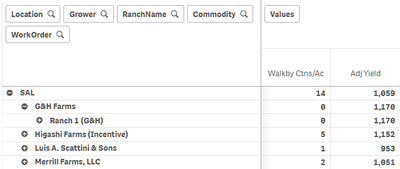App Development

Announcements
QlikWorld 2022, LIVE in Denver CO., May 16-19, 2022. REGISTER NOW TO RECEIVE EARLY BIRD PRICING
cancel
Showing results for
Did you mean:Creator III

Sum formula is not working correctly in pivot table

I have the following formula for Adjusted Yield in my pivot table:

If(Sum(WalkbyQty) > 0, (Sum(EU_RecQty) / Sum(Acres)) + (Sum(WalkbyQty) / Sum(Acres)),(Sum(EU_RecQty) / Sum(Acres)))

In my rows, I have five dimensions for drill down.  The Adjusted Yield formula does not calculate correctly at the top two levels; however, if I drill down further, then the formula appears to work correctly.  I have a feeling this formula might require more refinement such as with the Aggr function, but I would like to get some feedback and suggestions please.  Thank you.

Example of incorrect calculation for Adj Yield:Drill down one level on G&H Farms and all numbers suddenly are calculated correctly:1 Solution

Accepted SolutionsMVP

@mikegrattan  Your data model looks complex and this is the reason why you are facing this issue. Issue is that you have null values for Commodity dimensions, so when you drill down to to lower level pivot does not show null values and calculates correctly. At higher level, sum function always sums entire set of values. To avoid this one workaround is below.

If(Sum({<Commodity={"*"}>}WalkbyQty) > 0, (Sum({<Commodity={"*"}>}EU_RecQty) / Sum({<Commodity={"*"}>}Acres)) +
(Sum({<Commodity={"*"}>}WalkbyQty) / Sum({<Commodity={"*"}>}Acres)),
(Sum({<Commodity={"*"}>}EU_RecQty) / Sum({<Commodity={"*"}>}Acres)))

I am not sure if this completely solve the issue, but if at other level null values comes up and you don't want to consider that then you may also need to include the condition for that dimension like eg.

Sum({<Commodity={'*'}, Rower={'*'}>}Acres)

12 RepliesMVP

@mikegrattan  one way is to use dimensionality to aggregate your measure at each level of dimensions using aggr.

=Pick(dimensionality(),
sum(aggr(If(Sum(WalkbyQty) > 0, (Sum(EU_RecQty) / Sum(Acres)) + (Sum(WalkbyQty) / Sum(Acres)),(Sum(EU_RecQty) / Sum(Acres))), Location)),

sum(aggr(If(Sum(WalkbyQty) > 0, (Sum(EU_RecQty) / Sum(Acres)) + (Sum(WalkbyQty) / Sum(Acres)),(Sum(EU_RecQty) / Sum(Acres))), Location, Grower)),

sum(aggr(If(Sum(WalkbyQty) > 0, (Sum(EU_RecQty) / Sum(Acres)) + (Sum(WalkbyQty) / Sum(Acres)),(Sum(EU_RecQty) / Sum(Acres))), Location, Grower, RanchName)),

sum(aggr(If(Sum(WalkbyQty) > 0, (Sum(EU_RecQty) / Sum(Acres)) + (Sum(WalkbyQty) / Sum(Acres)),(Sum(EU_RecQty) / Sum(Acres))), Location, Grower, RanchName, Commodity)),

sum(aggr(If(Sum(WalkbyQty) > 0, (Sum(EU_RecQty) / Sum(Acres)) + (Sum(WalkbyQty) / Sum(Acres)),(Sum(EU_RecQty) / Sum(Acres))), Location, Grower, RanchName, Commodity, WorkOrder)))Creator III
Author

Hello Kushal,

I'm still seeing strange behavior after using your formula (adjusted to add a comma after "dimensionality()").  I'm only getting the right numbers after drilling down to level 3 in the Rows section.

Any suggestions?MVP

@mikegrattan  make sure that you are using actual field name in Aggr function. For eg. I have written Location, but in your case field name might be LOCATION_NAME. It will be hard to tell what's going wrong without looking into your data.Creator III
Author

There was one field name that had to be changed; Commodity should be CommDesc (Commodity is a valid field name, but CommDesc is being used in the pivot table).  I have changed the formula to use CommDesc, but there is still an aggregation issue at level one (Location) and level two (Grower).  After I drill down so level 3 (Ranch) is showing, the numbers are good.MVP

@mikegrattan  would you be able to share sample app? with expected output at level 1 and 2?Creator III
Author

I will make a sample version of the app and post it later today.  Thank you.Creator III
Author

Here's a sample copy of the application. I've reduced the amount of data and removed all unnecessary sheets and charts; only the pivot table is included, and I've modified it so only the fields involved in the formula are in the table.  With those modifications, it appears the formula you suggested is showing correct numbers after you drill down to level two. I'm not sure why it behaves this way, so any insight you can provide will be very appreciated.  Thank you.MVP

@mikegrattan  in your sample what should be the value at level 1? Could you give one example?Creator III
Author

If you filter on Year = 2021, CropType = LTC, and Location = SAL, the Adj Yield value at level one (SAL) shows up as 689.  If you drill down to level 3 to show Ranches under a Grower, the value at level one changes to 1064 which is the correct number. Note that the Adj Yield column is my original formula and the Adjusted Yield column is your suggested formula.Tags
Community Browser